0 Cart
You have items in your cart
You have 1 item in your cart
Total

# How to teach multiplication facts: proven strategies in special education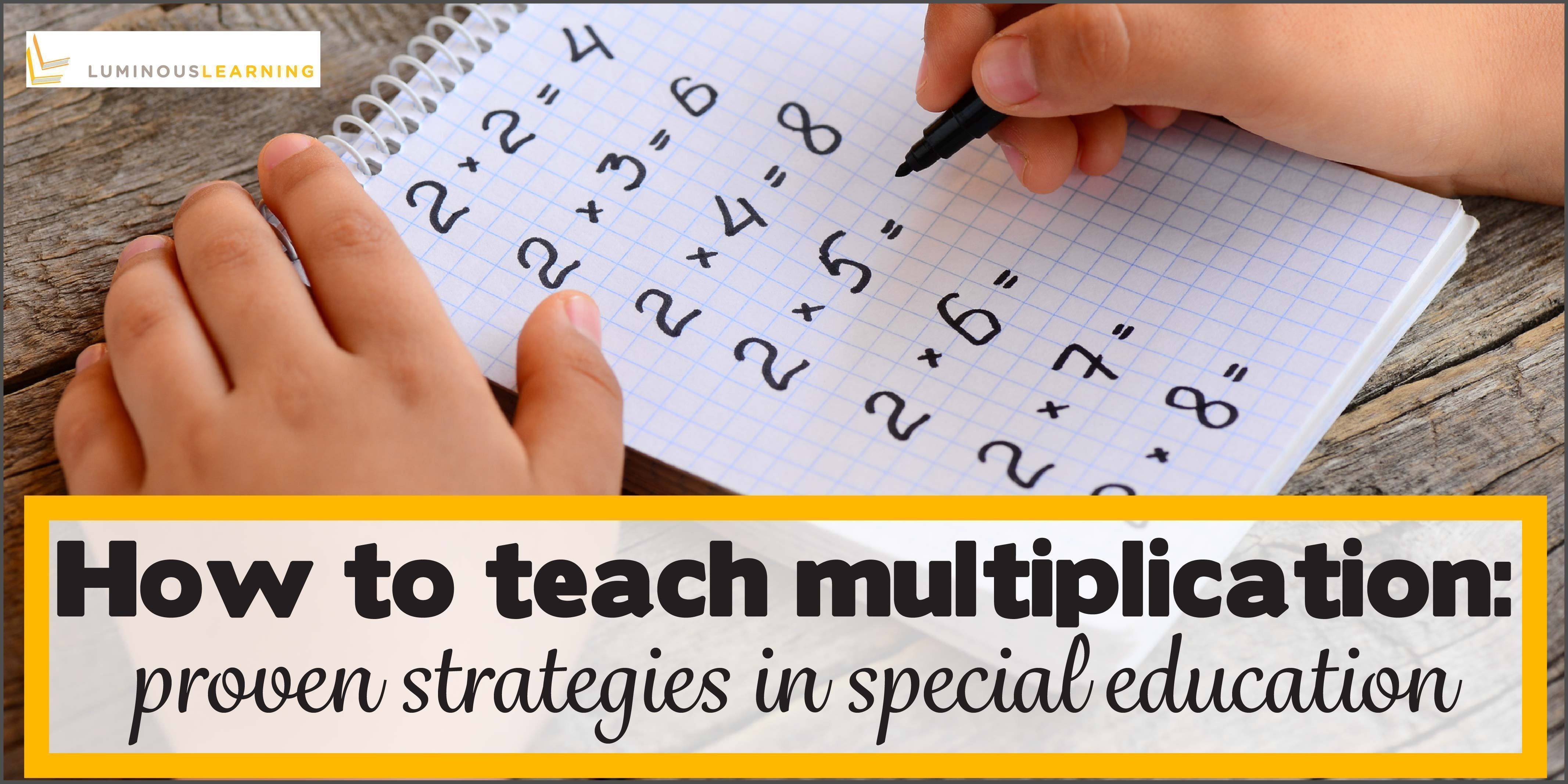Those days of forgetting their math facts?

Staring blankly at a multiplication problem?

Adding or subtracting when they should be multiplying?

Done. Gone. Over.

Those days are in the past!

Today we’re going to learn how to teach multiplication facts to our students with disabilities so that they build conceptual knowledge and retention.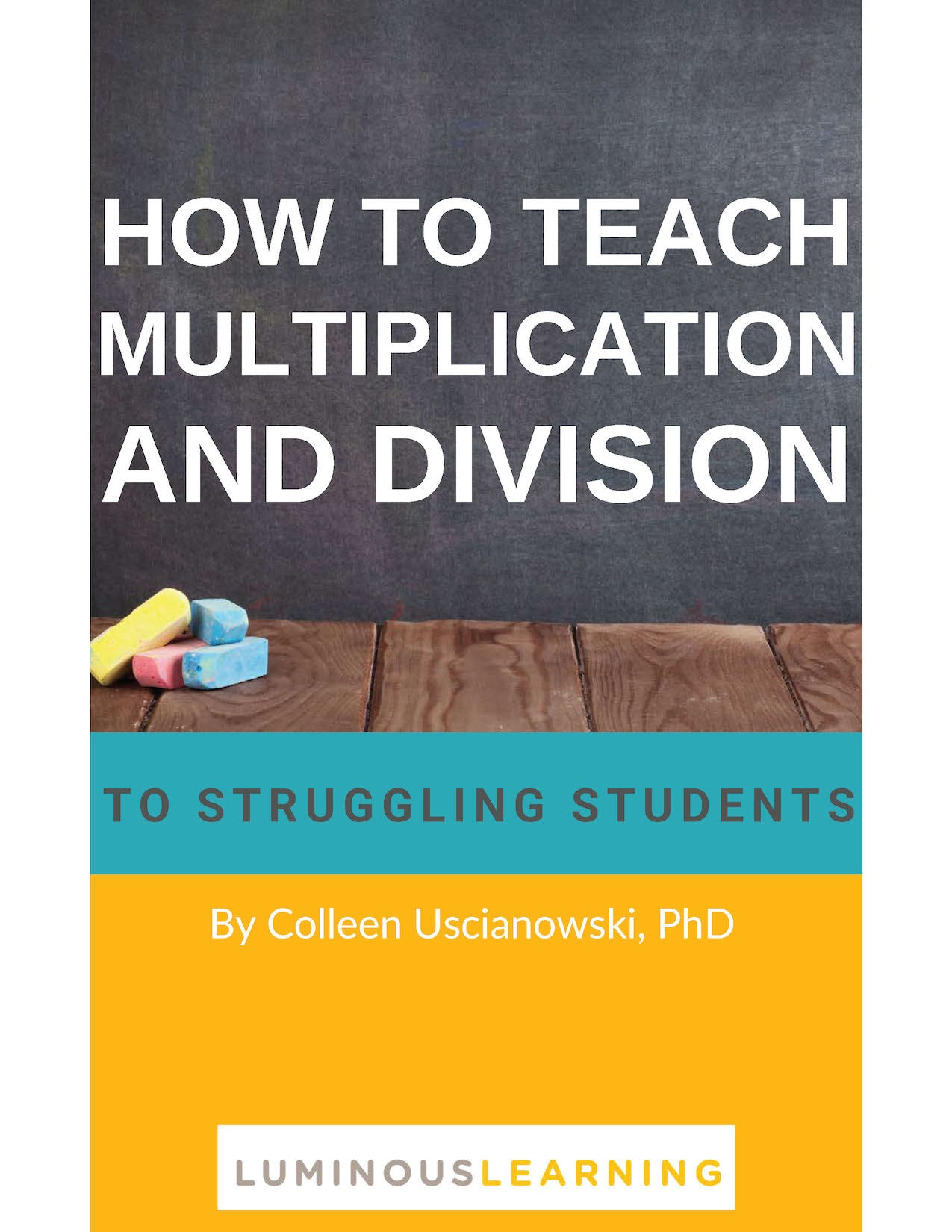# How to Teach Multiplication and Division ebook

Get your FREE 20+ page guide to teaching multiplication in the special education classroom + printable activities!

## The wrong way to teach multiplication facts to special education students

When I started out in the classroom, I couldn’t figure out why I would spend so much teaching multiplication facts one day just to have students come into class and forget them the next day!

Not only did they sometimes have trouble recalling the right answer to a multiplication problem, but they’d forget what it means to multiply.

For example, let's say they're solving for 6 x 5. Instead of making 6 groups of 5 counters, they would add 6 + 5. It seemed like all the teaching strategies I tried weren’t working.

Those flashcards? How about those timed multiplication drills? If your students don’t already have a strong conceptual knowledge of multiplication, throwing fact after fact at them just won’t work. In fact, it can actually harm them by increasing their math anxiety. And it sends the message that math is about getting the right answer quickly.

Read more about why fact fluency doesn’t involve speed or memorization.

If I could wave my magic math wand and make one thing disappear from classrooms everywhere, it would be those timed drills and rote practice.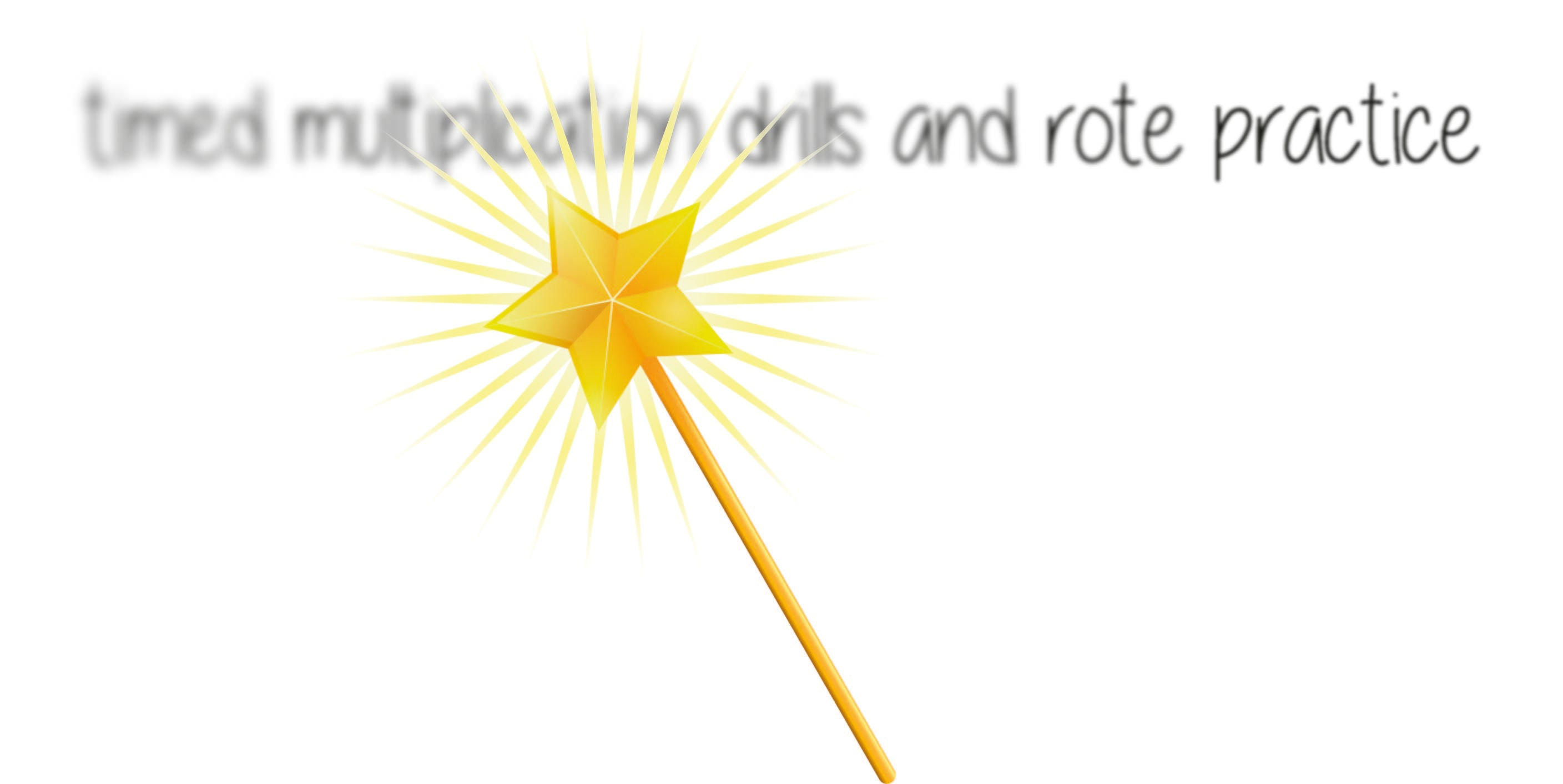### Recalling is not understanding

I now realize that recalling facts is different from understanding them and we need to use different strategies to promote retention and understanding. One reason I failed to help my students was because I wasn’t matching my math instructional strategies with where my students were in their development of multiplication knowledge.

Alright, so those were the things I did wrong back then. But what would I do differently today? I want to share the right way to teach multiplication to students with math learning disabilities.

## The right way to teach multiplication facts to special education students

Back in this post, we talked about the 4 stages of fact mastery.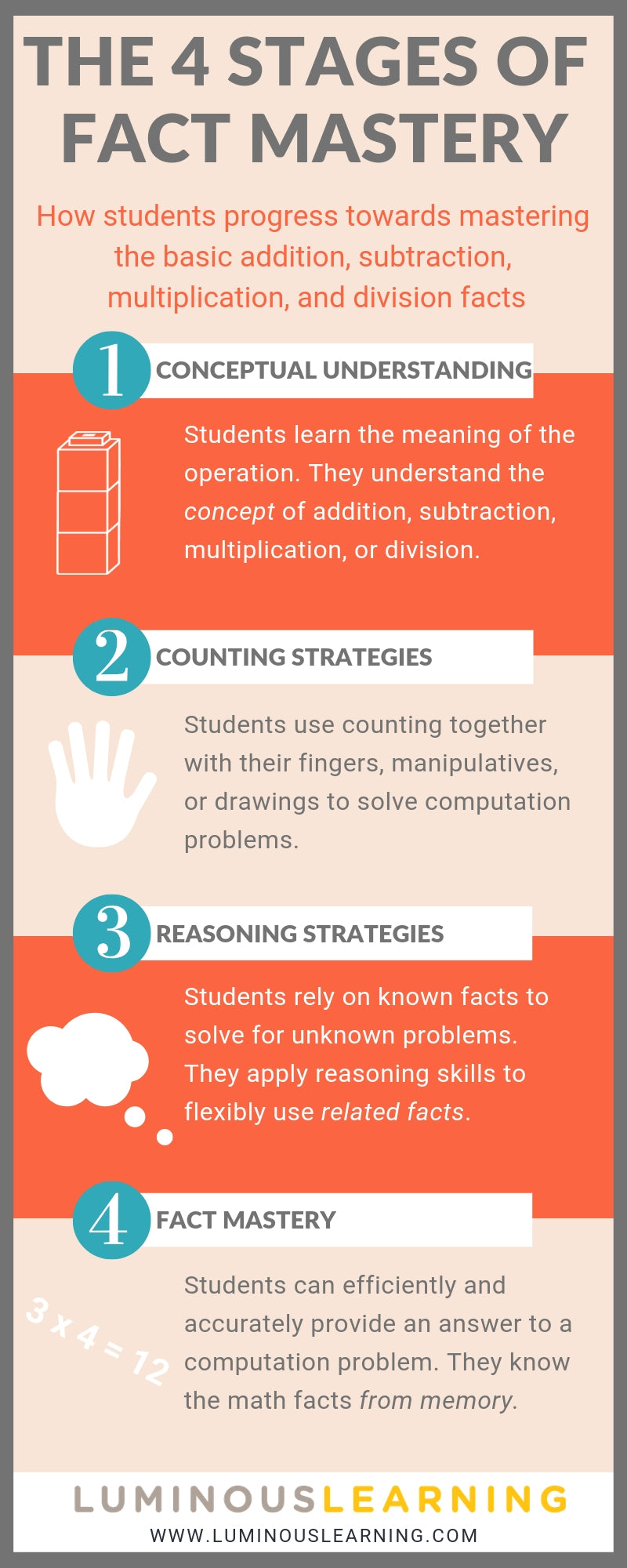Another way to think about students’ development of multiplication facts along a Concrete-Representational-Abstract continuum.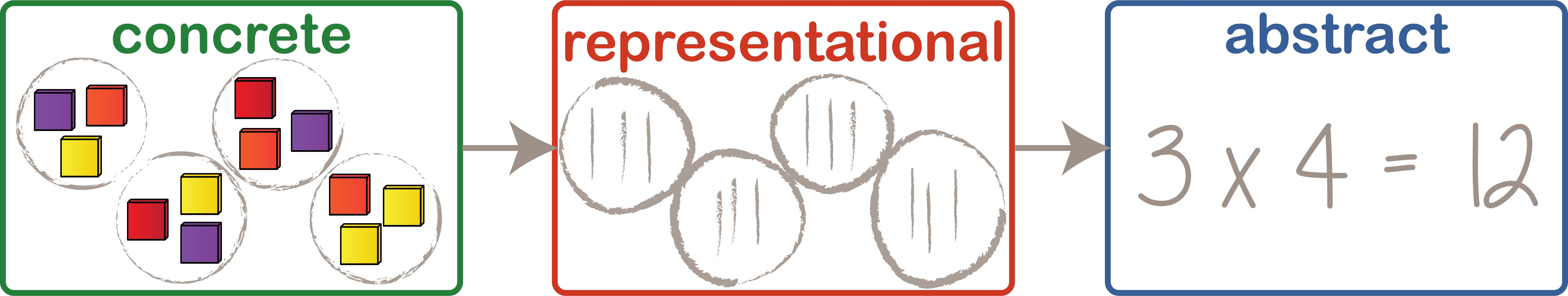### Concrete

In the beginning stages of learning to multiply, students need practice solving scenarios involving multiplication using concrete manipulatives, such as counters and unifix cubes. Students in the concrete phase are gaining conceptual understanding and practicing their counting strategies.

### Representational

After you spend time teaching multiplication problems using concrete materials over time, you can encourage students to use drawings or diagrams to solve multiplication problems. These drawings are similar to the manipulative in that they model the problem, but they are more abstract and therefore require more mathematical thinking.

Students in the representational phase are solidifying their conceptual understanding and counting strategies while beginning to apply more advanced reasoning skills to solve multiplication problems.

### Abstract

After students have adequate practice first solving multiplication problems using concrete manipulatives and then drawings or diagrams, they will be ready to solve multiplication equations. This type of problem is abstract because it involves symbols that students need to interpret.

Students in the abstract phase have already gained a strong conceptual knowledge of multiplication. They are practicing their reasoning skills, which leads to fact mastery.

Students in each of these phases need a different kind of instruction in order to improve their multiplication skills. Before you can choose an appropriate teaching strategy, you’ll need to administer a math assessment to find out where they are along the path towards fact mastery.

Learn exactly how to assess your students' multiplication and division skills in my FREE online course! Read more and sign up here.

Next, depending on the results of your assessment that tell you where your special education students are, they will need different math instructional strategies.

## Here are teaching strategies to use for each stage of multiplication development:

### How to teach multiplication facts using concrete strategies that build conceptual understanding

Forget about multiplication equations for a moments and introduce the concept of multiplication through word problems and scenarios. For example:

Lily is putting candles on a cake for her Dad’s birthday. She makes 6 rows of candles with 7 candles in each row. How many candles does Lily use?

Let students solve the problem in any way they know how. Engage the class in math talks about the various ways they solved each problem.

You can also have math talks about examples of multiplication we see all around us. This helps your students see math as something relevant to their lives, not as abstract numbers that only exist during math class. For example, what conversations would you have with your class about this photo?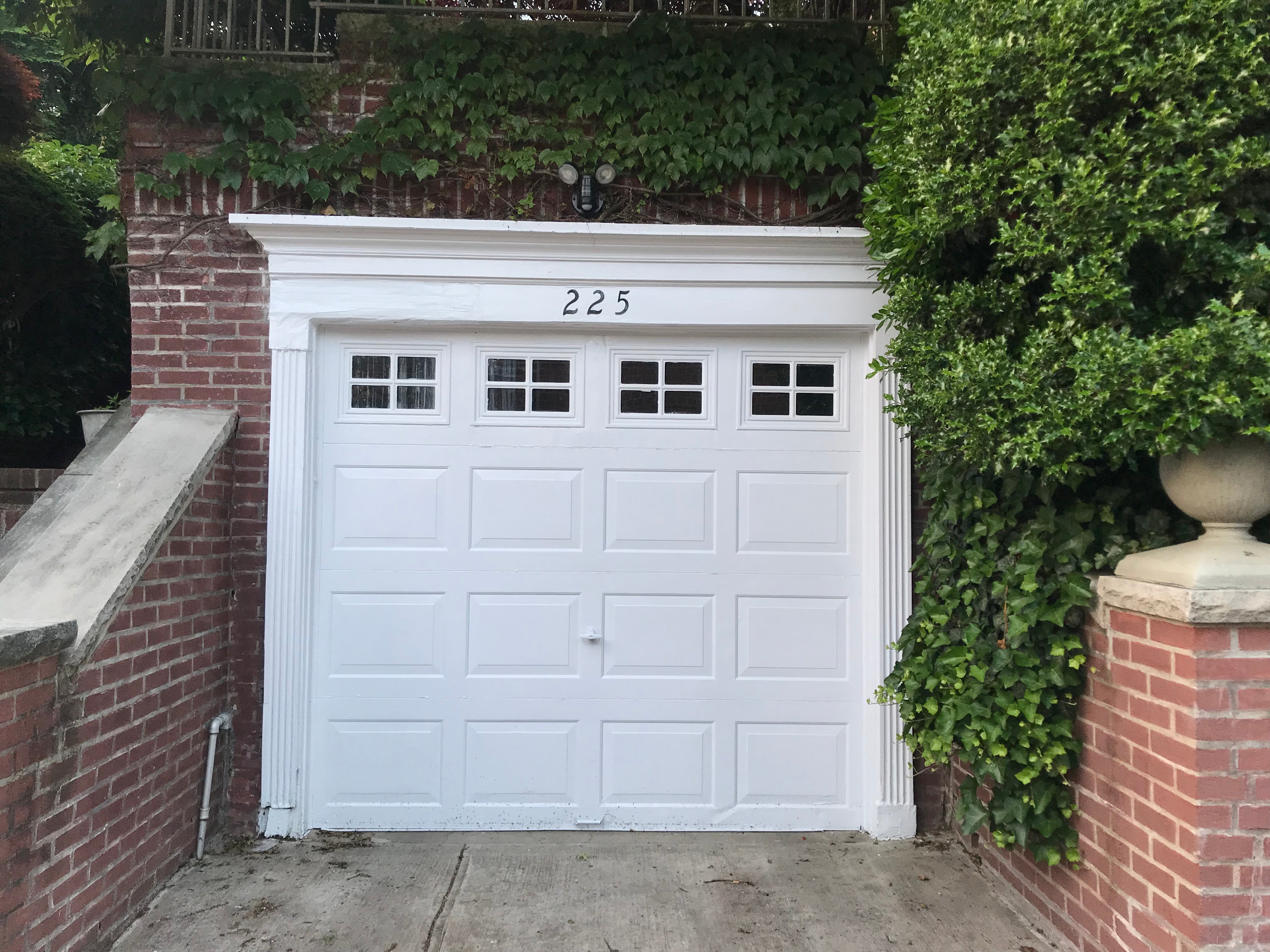Model and think aloud about how you would solve multiplication problems using manipuatives. At the concrete phase, it's all about the manipulatives! As you model problems and encourage students to use manipulatives, make sure that you show different representations of multiplication, such as:

#### arrays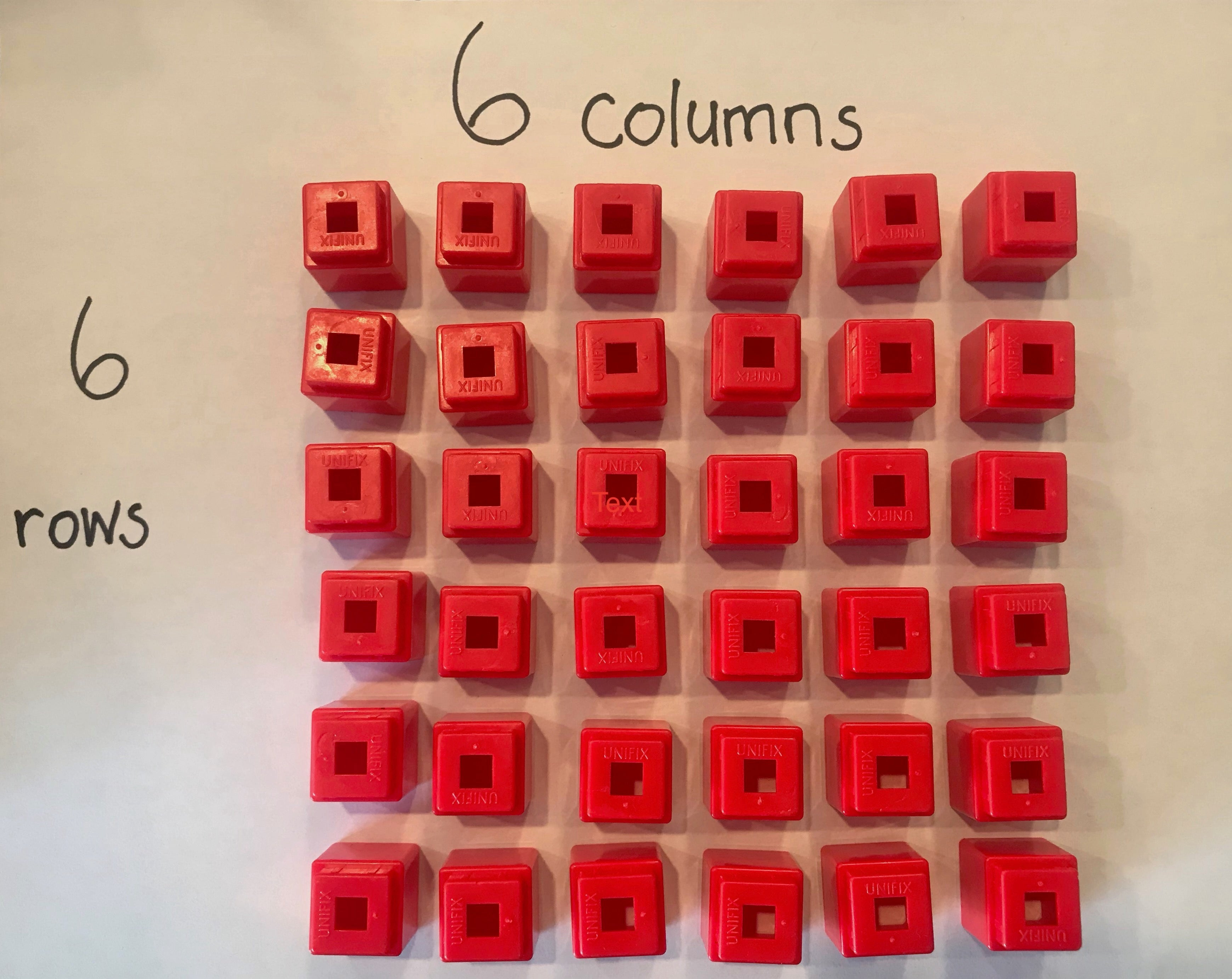#### equal groups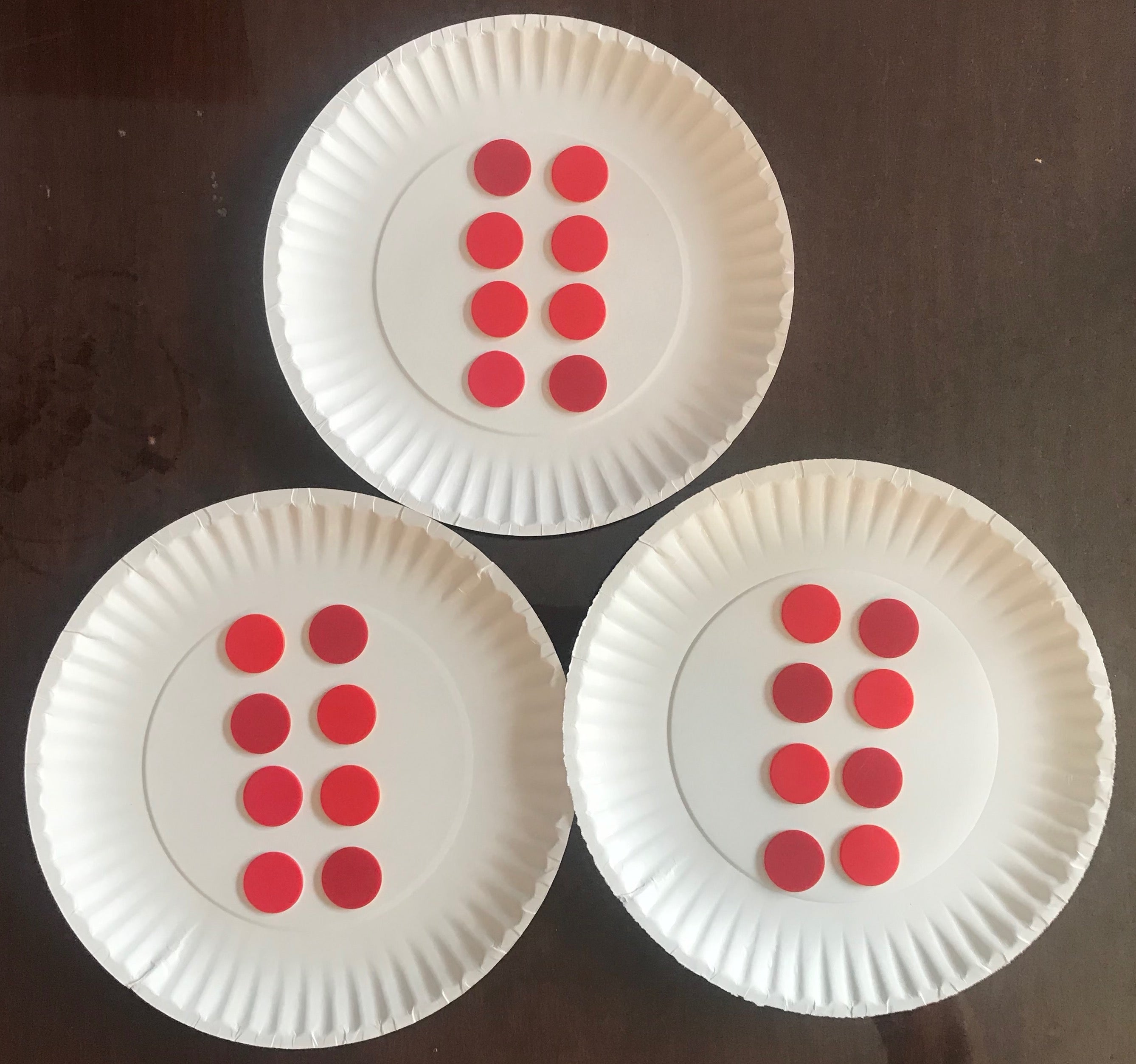#### scalar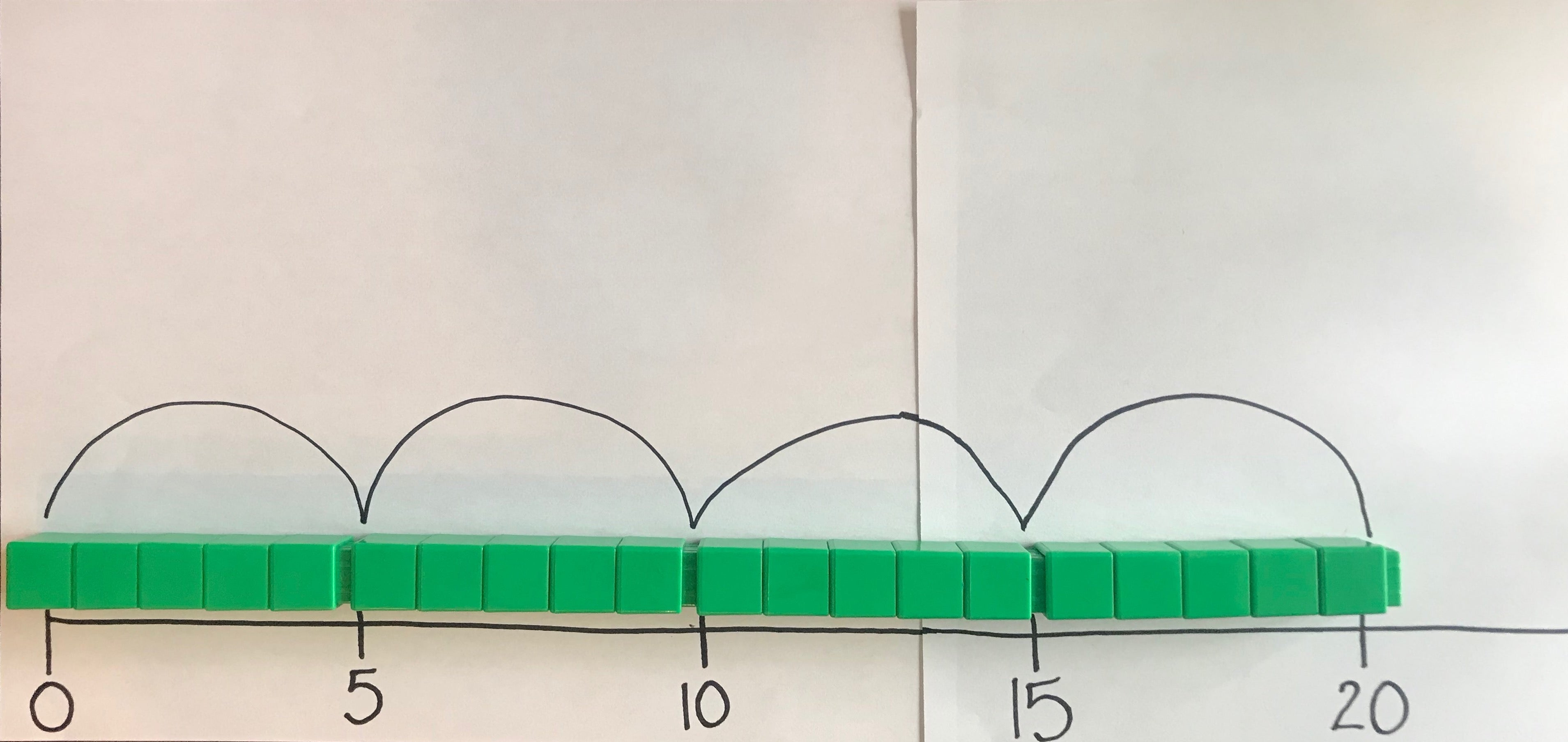### How to teach multiplication facts using these teaching strategies at the representational phase

Keep using word problems, but begin to translate the problem into equations. For example:

Lily is putting candles on a cake for her Dad’s birthday. She makes 6 rows of candles with 7 candles in each row. How many candles does Lily use?

"I notice Lily made 6 rows, so I can draw 6 rows with 7 in each row. 6 rows of 7 makes me think of 6 times 7."

Continue using math talks, but model how to translate the picture into a drawing or diagram.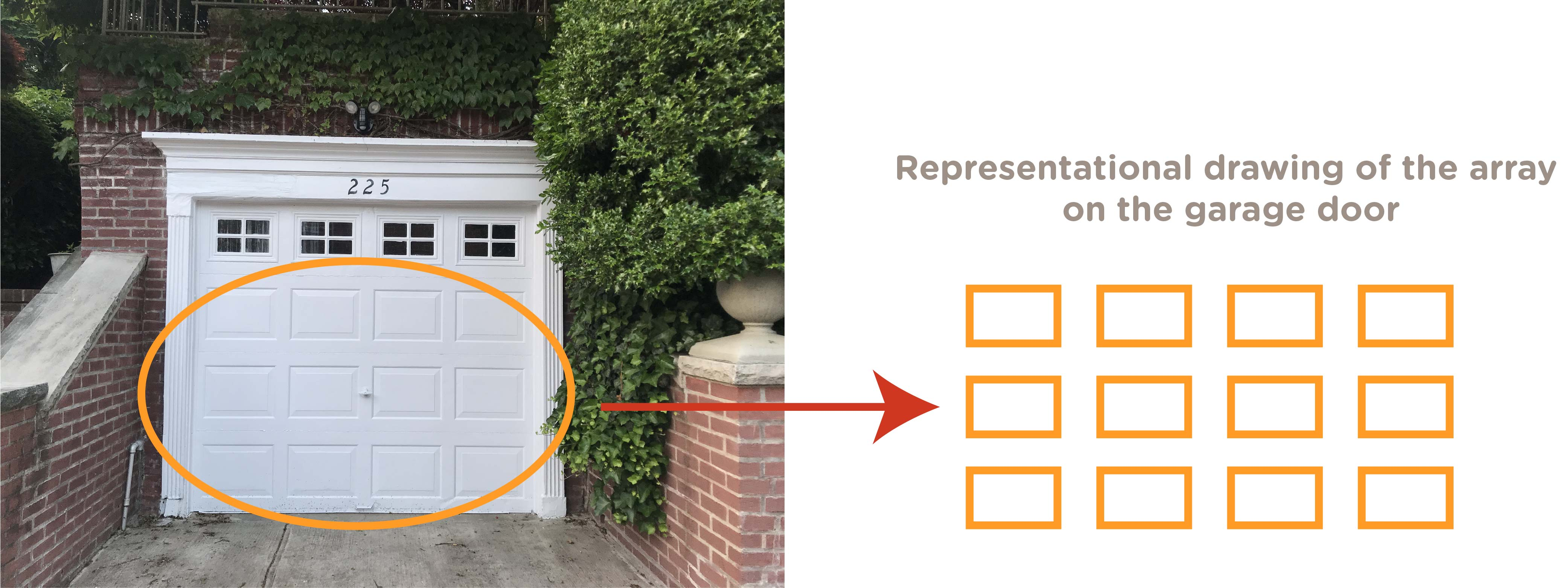Model how to solve multiplication word problems by drawing a picture or diagram. Similar to the concrete phase, show different representations of multiplication using:

### arrays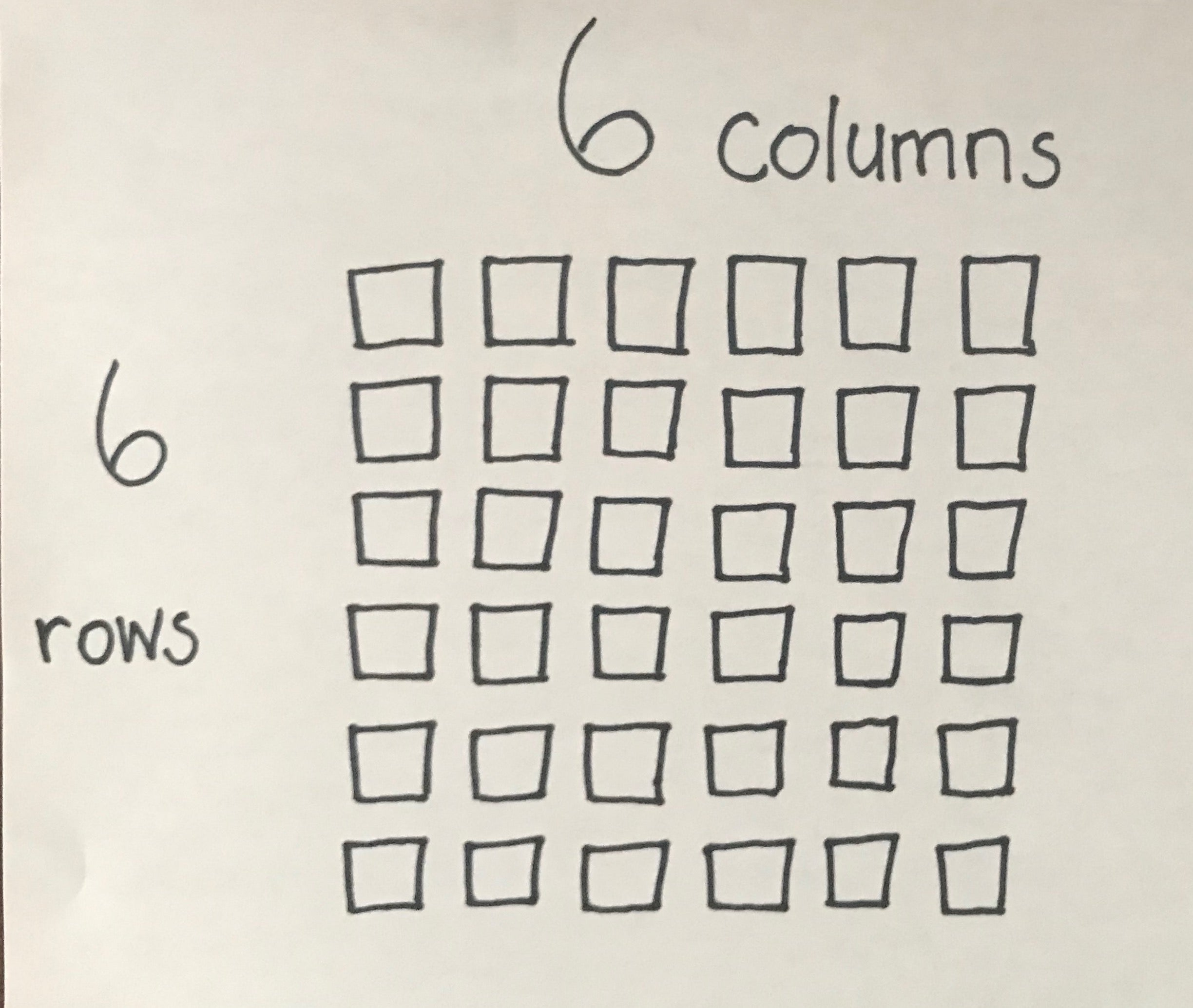### equal groups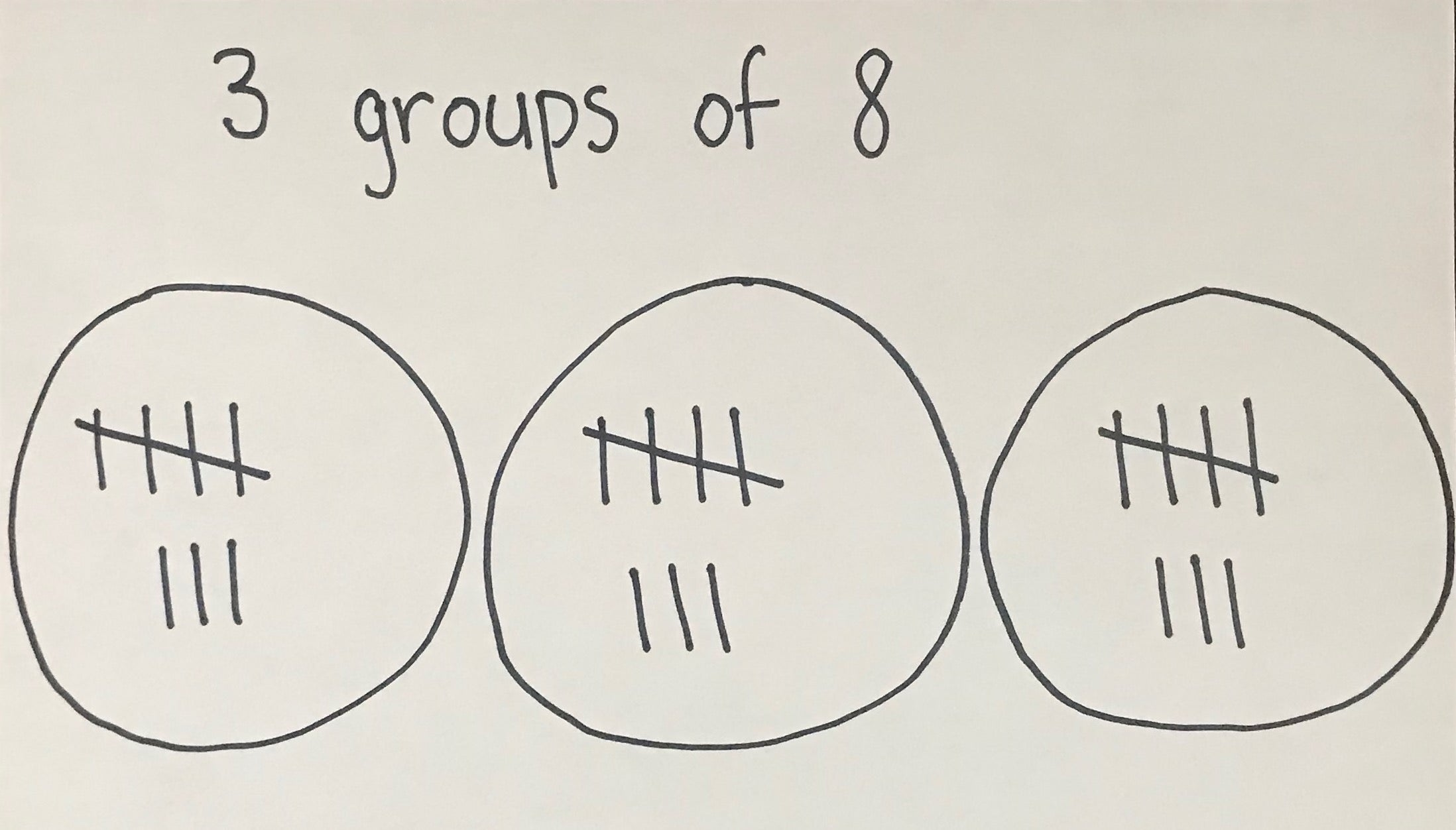### scalar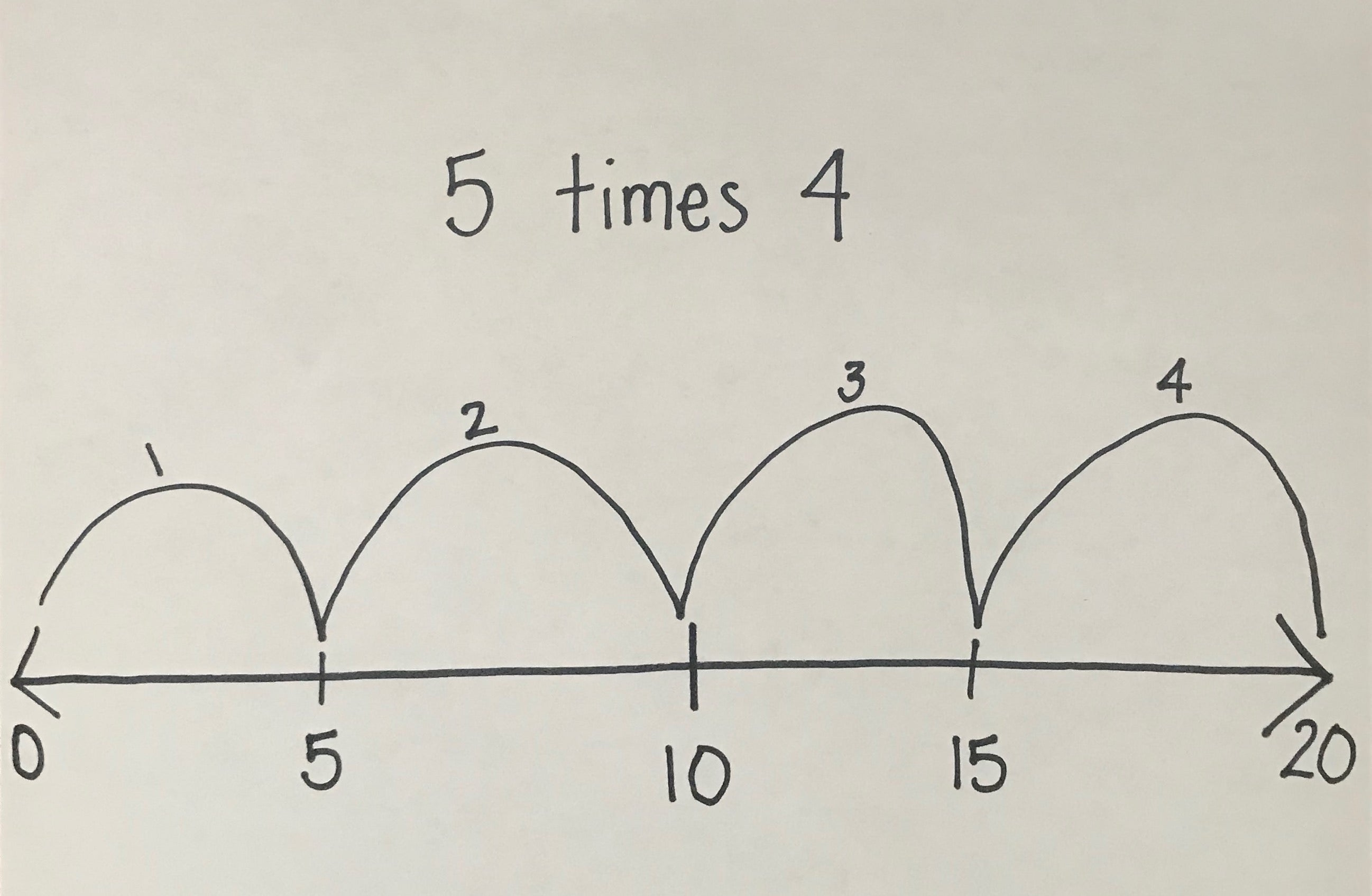After students have played multiplication games and activities and practiced solving problems with manipulatives and drawings, they will have strengthened their conceptual understanding of what it means to multiply.

Now it’s time to work on building procedural fluency so that students have more efficient ways to solve problems. Students will be ready to solve more abstract problems dealing with just the symbolic numbers.

### How to teach multiplication to promote an abstract understanding of math facts

At this stage, we’re working towards fact mastery. Some math instructional strategies you can use include:

Translating word problems directly into abstract symbols and numbers.

Lily is putting candles on a cake for her Dad’s birthday. She makes 6 rows of candles with 7 candles in each row. How many candles does Lily use?

"6 rows and 7 columns means 6 x 7."

Some students will be able to recall these multiplication facts from memory. However, many of our students with math learning disabilities will struggle with memory issues. They won’t be able to quickly and efficiently retrieve facts from memory.

And that’s ok!

Instead, you can teach them to rely on known facts. For example: Multiplying any number x3 is the same as multiplying the number x2 plus adding one more group.

2 x 3 = 2 x 2 + 2

3 x 3 = 3 x 2 + 3

4 x 3 = 4 x 2 + 4

5 x 3 = 5 x 2 + 5

You can double the first number then add another group.

At this stage, after students have a strong understanding of what it means to multiply, you can also have students practice skip counting to help improve their memory for multiplication facts. Choose one number to practice at a time, for example, skip counting by 3s. Practice skip counting to a chant or a rhythm. Clap or stomp along to the rhythm. Keep it anchored to something concrete, such as the number line, so that students aren’t blindly memorizing a string of words.

### WRAPPING IT UP...

Are you ready to wave your magic math wand and make those timed drills and rote practice disappear from math classrooms everywhere?

As we just saw, there are better ways to teach multiplication to students with math learning disabilities. These instructional strategies help students develop a strong conceptual understanding of multiplication facts until they eventually reach fact mastery.

#### Now, let’s chat in the comments: What are some of your strategies for teaching multiplication?# How to Teach Multiplication and Division ebook

Get your FREE 20+ page guide to teaching multiplication in the special education classroom + printable activities!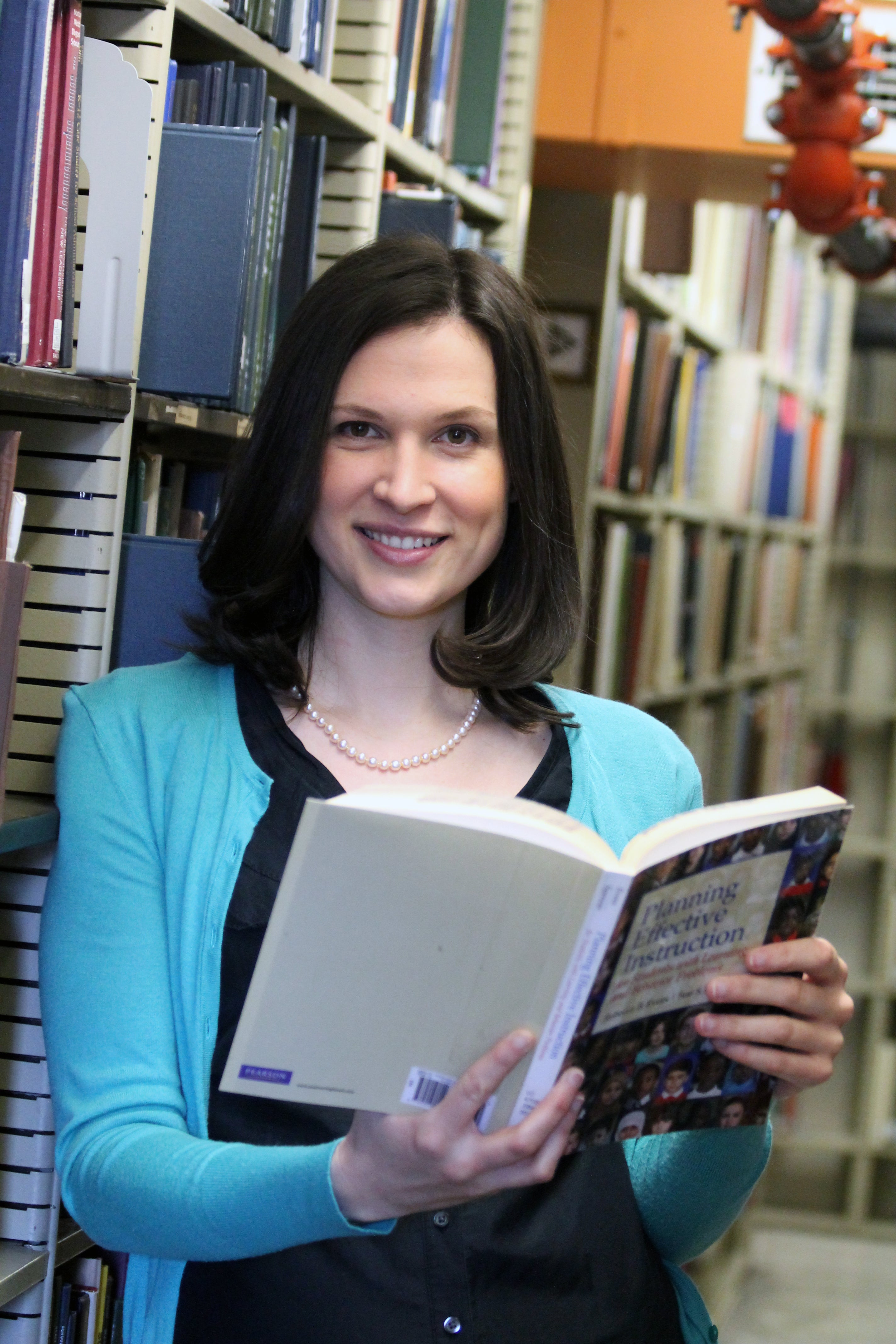### Hi, I'm Colleen

I believe math is for everyone and even our most struggling students can grow their math minds. I help special education teachers improve their math instruction and send their students soaring to new heights!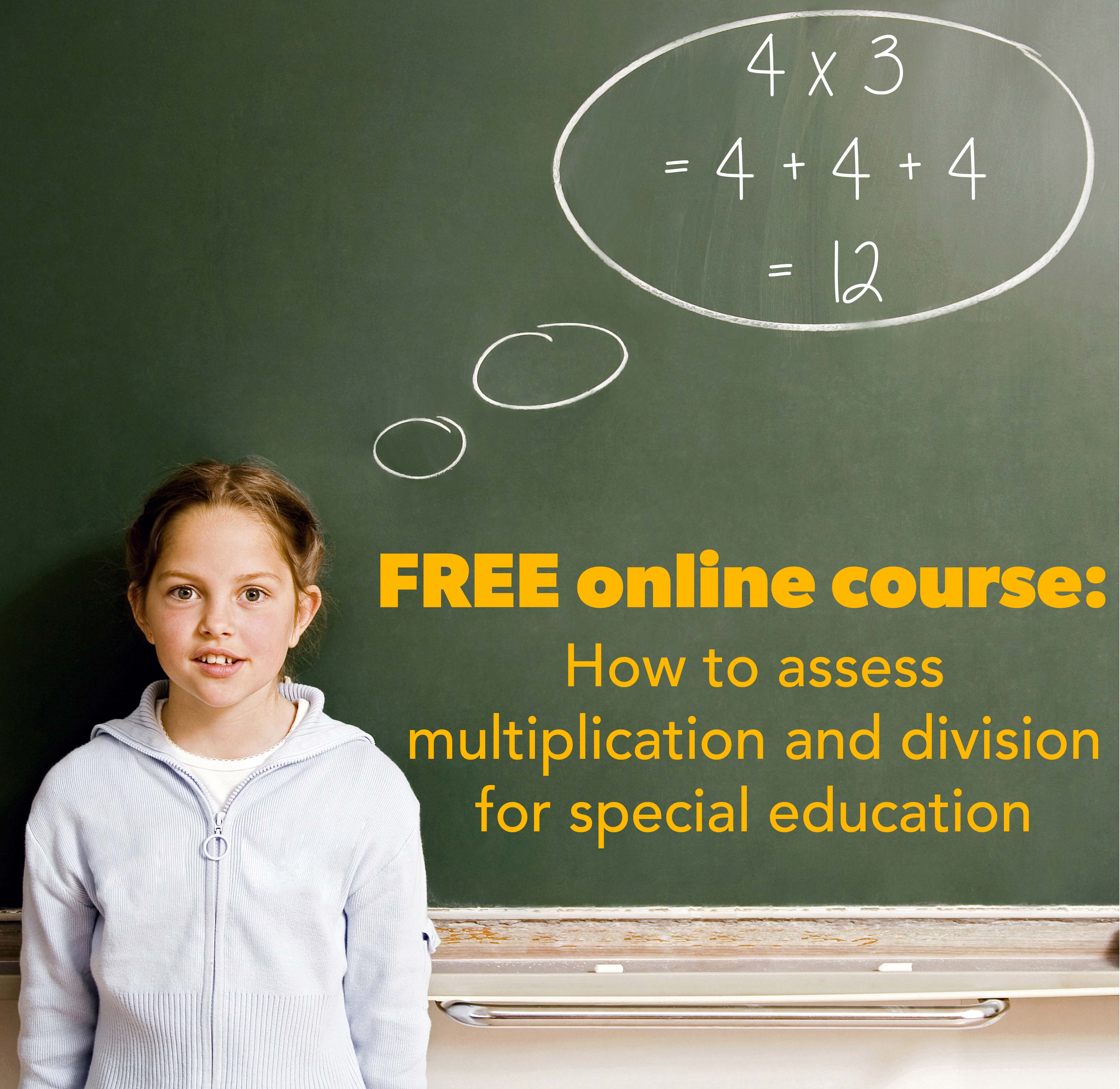## I want to learn about...

##### Math learning disabilities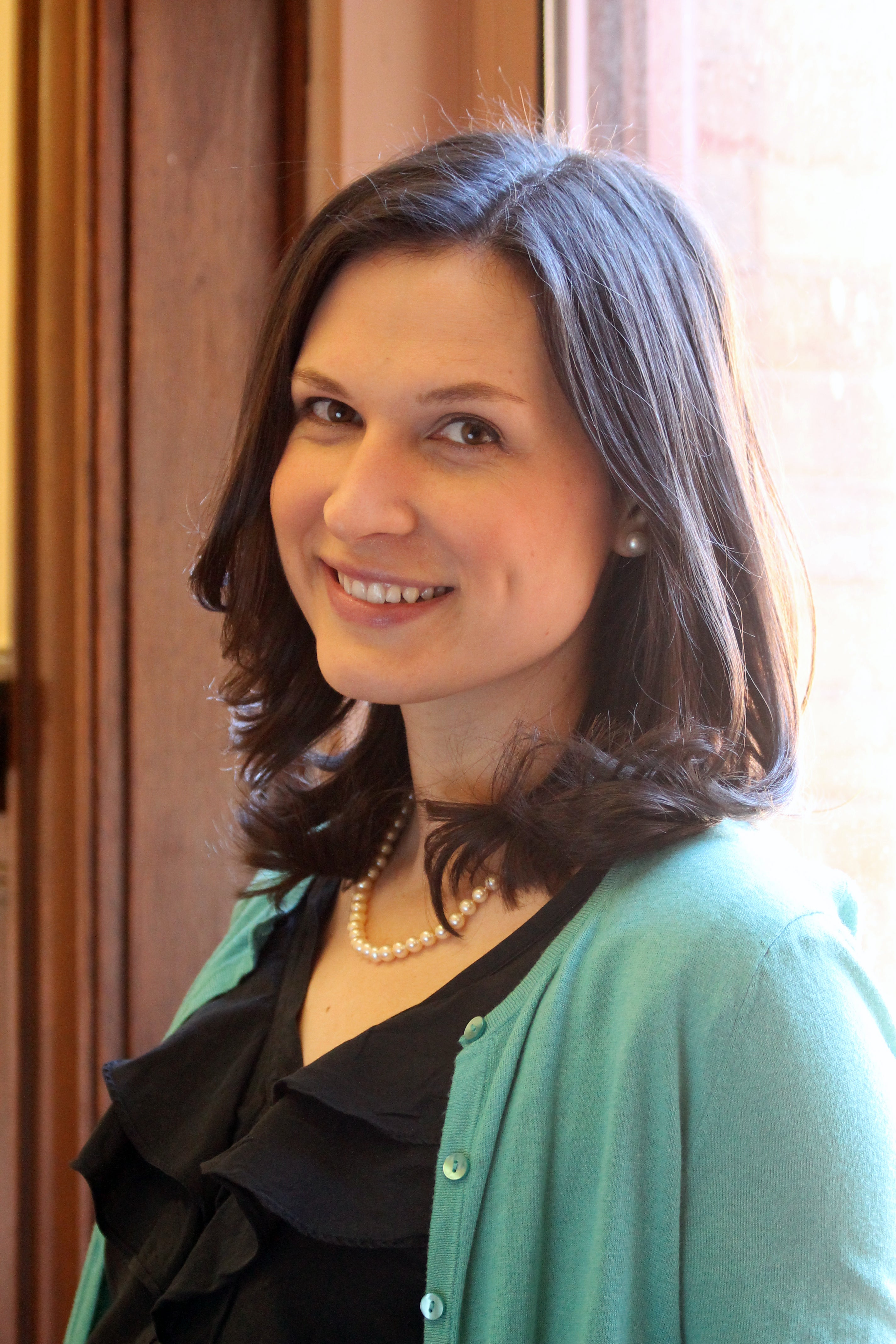### AUTHOR: DR. COLLEEN USCIANOWSKI

Colleen teaches courses for special education teachers and creates curricular resources for special education students. She was a special education teacher who received her PhD in cognitive psychology and bases her work on cognitive science principles. She's passionate about changing the way we teach special education students and deeply believes that math is for everyone!